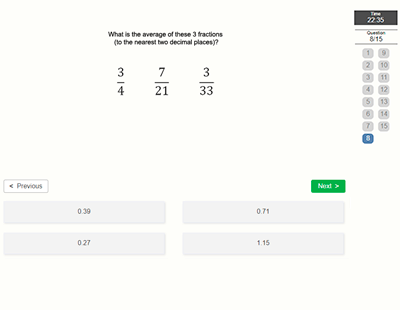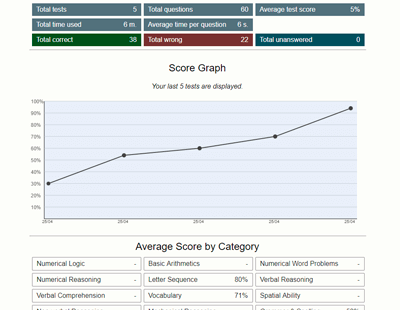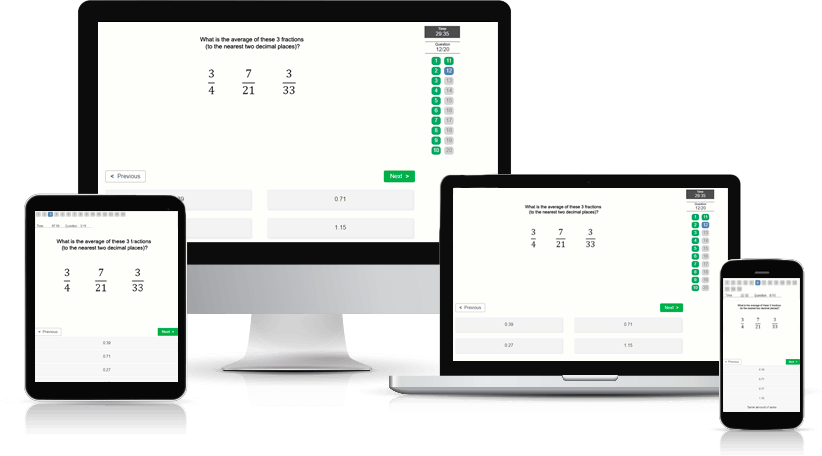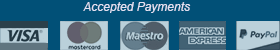﻿ Numerical Logic Test - Aptitude-Test.com

# Numerical Logic Test

Numerical Logic is also commonly called number series. In these test questions you will be presented with a series of numbers with one number missing, you will then need to find the missing number. Numerical Logic tests assess your logical thinking ability.

What questions can I expect?
In a numerical logic test you can expect questions in the following format:

Example question:
Find the missing number.
2, 4, 8, ?

A) 10
B) 6
C) 16
D) 12

Explanation:
In the series we can see that the number is doubled by every step to the right, therefore the correct answer is option C, 16.

Where are Numerical Logic tests used?
Numerical logic tests are frequently used and the question type is very common as part of pre-employment aptitude tests, especially tests assessing Cognitive Ability.## Practice Numerical Logic Test

Try a free Numerical Logic Test.
This free practice test contains 6 test questions and has a time limit of 6 minutes.

##### Would you like to improve your test score? Practice smart with a Test Prep Account.

Practice on 130 Numerical Logic and a total of 530 numerical aptitude test questions with detailed description and score statistics.

The many features of a Test Prep Account:

• Preparation software developed under a didactic and methodical perspective
• High-quality practice test questions
• Clearly explained solutions
• Accessible on all devices
• Detailed score data and progression charts
• Reference scores to compare your performance against others
• Training Assistant
• Practice mode (feedback after every question and no time limit)